Are you excellent at maths?

If you have tried the Number Lines and Solution Sets quiz you will be familiar with the idea of a horizontal number line, which can be used to define the position relative to zero. But what if we want to add in a second dimension, like on a map? Not only do we want know how far east or west of a central point something is, we also want to know how far north or south it is. To do this we introduce a second number line, at right angles to the first. These two lines, called AXES, cross each other at zero. This point is known as the ORIGIN. See how well you can work your way around a pair of axes in this GCSE Maths quiz.

We use COORDINATES to define a position relative to the origin. These are written inside brackets, and are separated by a comma.

The first number indicates the distance from the origin in the x-direction (horizontally), and the second number is the distance from the origin in the y-direction (vertically). Just as on a standard number line, a negative value indicates left or below the origin.

The axes form four distinct areas, known as QUADRANTS. When you first started working with coordinates you would have had only positive values of x and y, and so would have been working in the first quadrant only. Continue in an anticlockwise direction to name the second, third and fourth.

Just as in so many other areas of maths, it’s a good idea to sketch out the situation when you are solving problems involving coordinates.

Question 1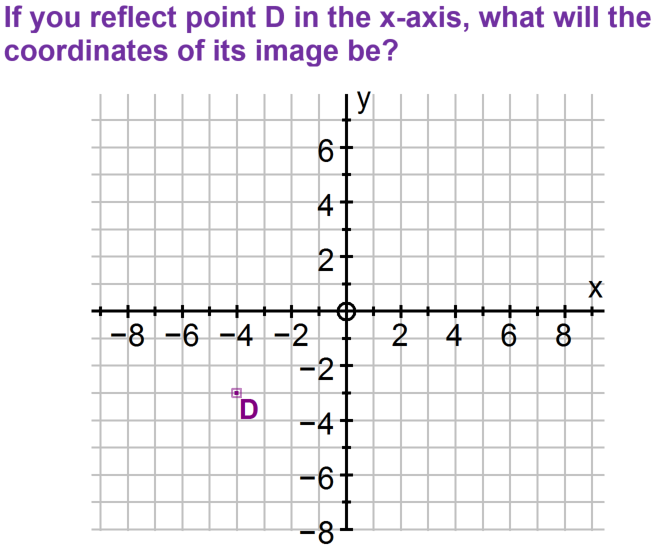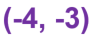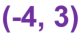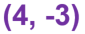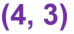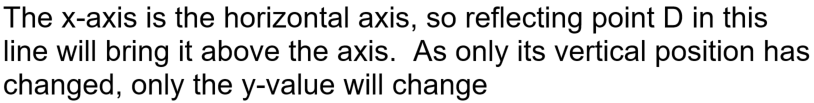Question 2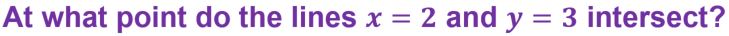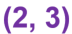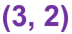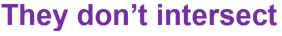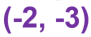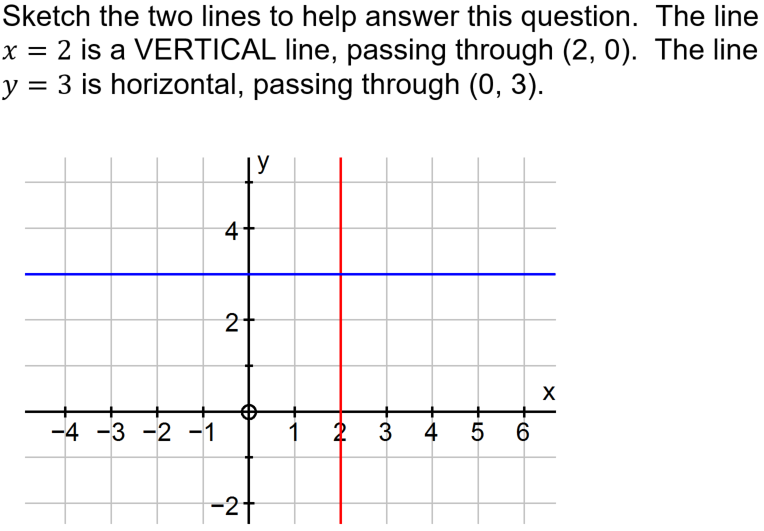Question 3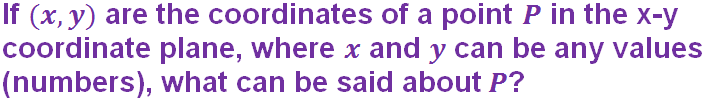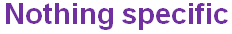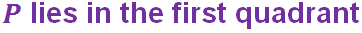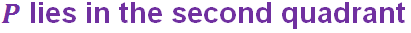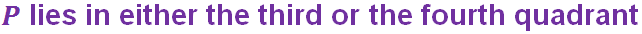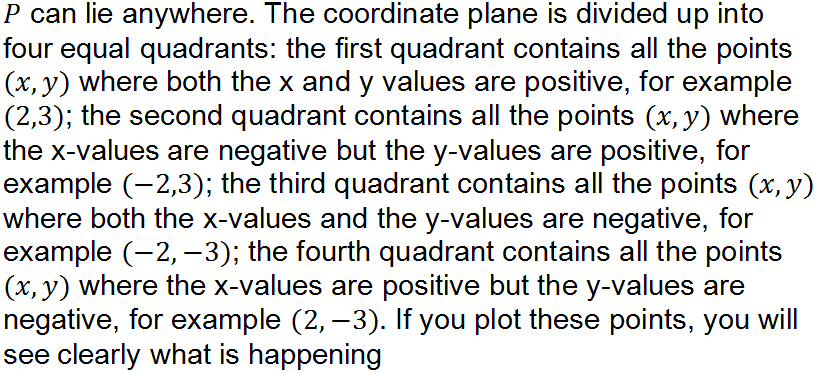Question 4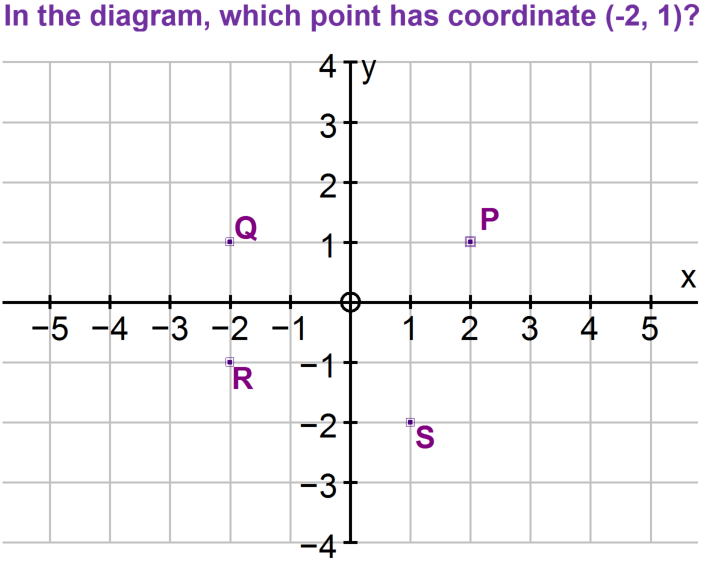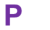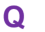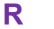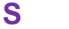Question 5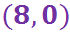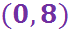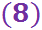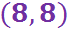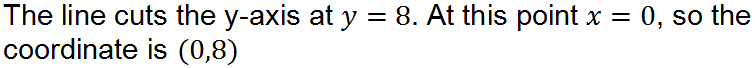Question 6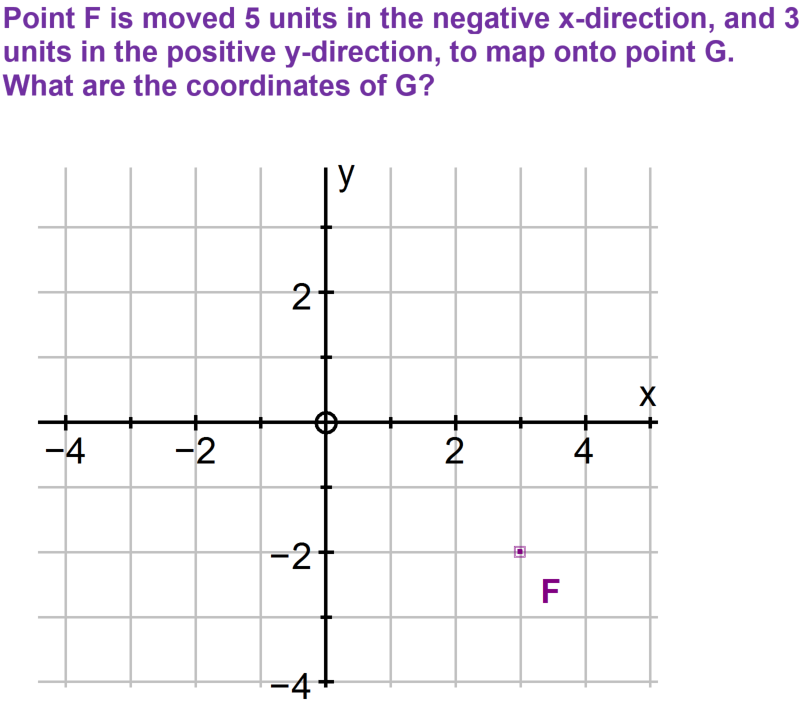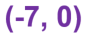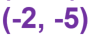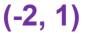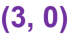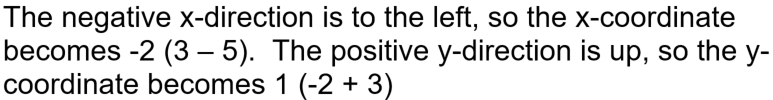Question 7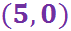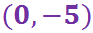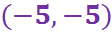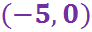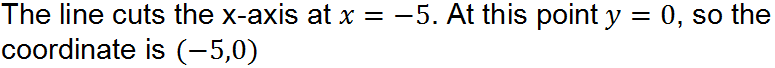Question 8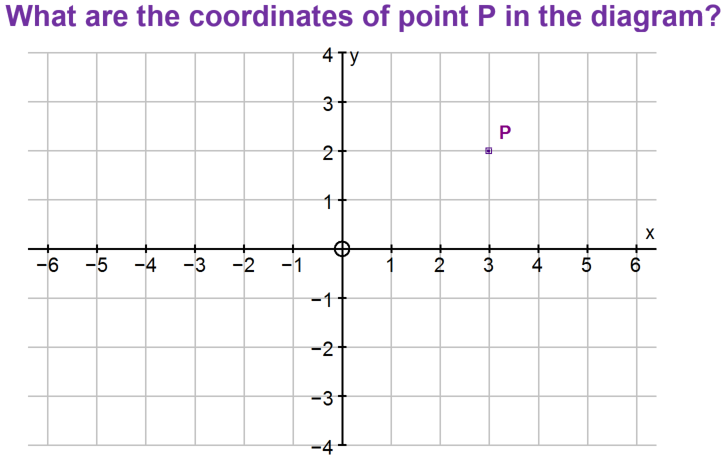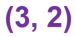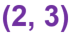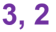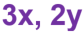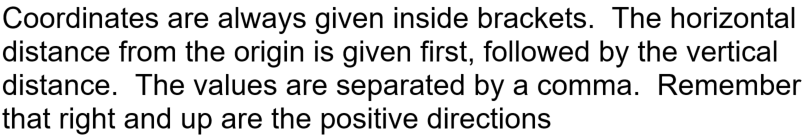Question 9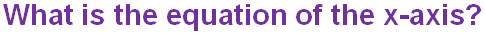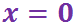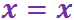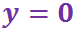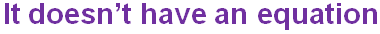Question 10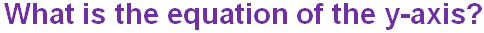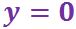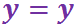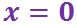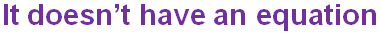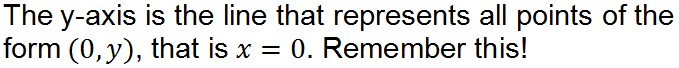Author:  Sally Thompson